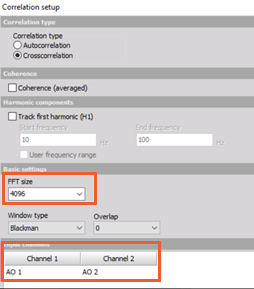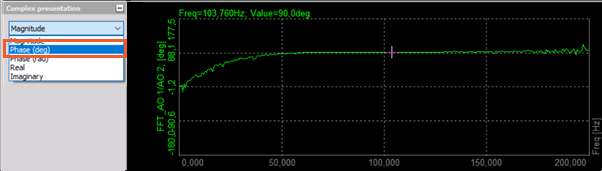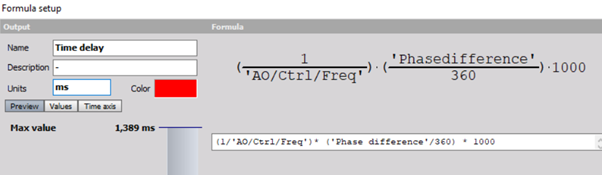## Description of the problem

How to calculate the time delay between two signals?

## Description of the solution

For time delay calculation between two sine signals a math Correlation will be used. For an example we will use sine signals with a frequency of 100 Hz and phase shift of 90°. The time difference between these two sine signals is 2.5 ms.In Correlation math choose crosscorrelation and select the two sine signals.The FFT results from the crosscorrelation can be viewed in the 2D graph. The MAX crosscorrelation value is at 100 Hz:Changing the complex presentation of the crosscorrelation to PHASE(deg) will show the result:Because the max peak of crosscorrelation is at 100Hz, we are interested in the phase at 100Hz. This can be calculated with the use of the formula:

Phase difference = angle('FFT_AO 1/AO 2'{100})*180/piPhase difference result:The time delay (in milliseconds) can be calculated from the phase difference and the frequency of the signal with the formula:

Time delay = (1/'AO/Ctrl/Freq')*('Phase difference'/360)*1000Time delay calculation: